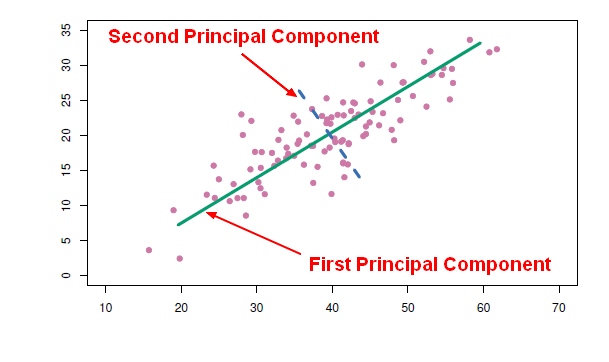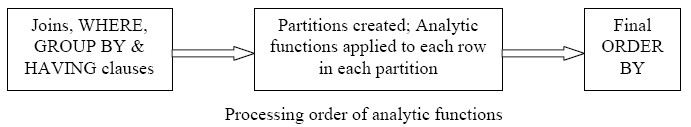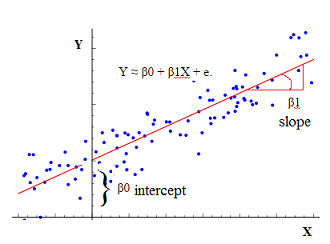# Statistics - Correlation (Coefficient analysis)

Correlation is a statistical analysis used to measure and describe the relationship between two variables.

The Correlations coefficient is a statistic and it can range between +1 and -1

• +1 is a perfect positive correlation. If the scores goes up for one variable the score goes up on the other.
• > 0.8 is a strong correlation
• > 0.4 is a high correlation
• > 0.2 correlate
• < 0.2 is not a strong correlation
• < 0.1 doesn't correlate
• 0 is no correlation (independence)
• -1 is a perfect negative correlation. If the scores goes up for one variable the score goes down on the other.

Correlation is used:

• mainly to describe relationship
• and then it's used for prediction that leads to regression because when two variables are correlated, then one variable can be used to predict the other variable. In other words, if the variables X and Y are correlated, a regression can be used to predict Y from X.

If two variables are correlated, X and Y then a regression can be done in order to predict scores on Y from the scores on X.

Correlation demonstrates the relationship between two variables whereas regression provides an equation (with two or more variables) which is used to predict scores on an outcome variable.

Positive correlation only means that the univariate regression has a positive correlation. In a multiple regression, the sign (positive, negative) is dependent of the other variables.

## Correlation does not imply causation

Correlation does not imply causation but correlations are useful because they can be used to assess:

## Type

There are several types of correlation coefficients, for different variable types

## Venn diagrams

Venn diagrams representation of a correlation between two variables X and Y.

Venn diagrams representing:

• All the variants in X,
• All the variants in Y
• And the overlap (the covariance). The overlap is can also be expressed as:

The degree to which x and y correlate is represented by the degree to which these two variance circles overlap. The correlation (degree|coefficient) is the systematic variance in Y that's explained by X.

The correlation is approaching:

• one for an high degree of overlap
• zero for no overlap

The residual is the unexplained variance in Y. Some of the variance in Y is explained by the model. Some if it is unexplained, that's the residual.

## Documentation / Reference

Discover MoreConsumer Analytics - Privacy

The purpose of data mining is to discriminate … who gets the loan who gets the special offer Certain kinds of discrimination are unethical, and illegal racial, sexual, religious, … But it...Data Mining - Principal Component (Analysis|Regression) (PCA|PCR)

Principal Component Analysis (PCA) is a feature extraction method that use orthogonal linear projections to capture the underlying variance of the data. By far, the most famous dimension reduction approach...R - Bootstrap

in R. Bootstrap lets you get a look at the sampling distribution of statistics, for which it's really hard to develop theoretical versions. Bootstrap gives us a really easy way of doing statistics when...R - Correlation

with: confidence interval df (degree of freedom) t-value p-value cor (Pearson's product-moment correlation)SQL Function - Window Aggregate (Analytics function)

Windowing functions (known also as analytics) allow to compute: cumulative, moving, and aggregates. They are distinguished from ordinary SQL functions by the presence of an OVER clause. With...Statistics

is a scientific discipline devoted to the study of data. is the art of extracting information from data. From Data to Information to Knowledge. No learning. lies lies, damned lies, and statistics....Statistics - (Regression Coefficient|Weight|Slope) (B)

The regression coefficients, the slopes, or, the B values represent the unique variants explained in the outcome by each predictor. For a simple regression: For a multiple regression: The regression...Statistics - (Univariate|Simple|Basic) Linear Regression

A Simple Linear regression is a linear regression with only one predictor variable (X). Correlation demonstrates the relationship between two variables whereas a simple regression provides an equation...Statistics - Assumptions underlying correlation and regression analysis (Never trust summary statistics alone)

The magnitude of a correlation depends upon many factors, including: Random and representative sampling Measurement of X and Y: Reliability of X and Y Validity of X and Y Several other assumptions:...Statistics - Correlation Matrix

From a raw matrix to a correlation matrix. A correlation matrix is a special matrix used in statistics. It is a square symmetric matrix. 3 columns (3 variables), 8 rows (8 individuals) ...